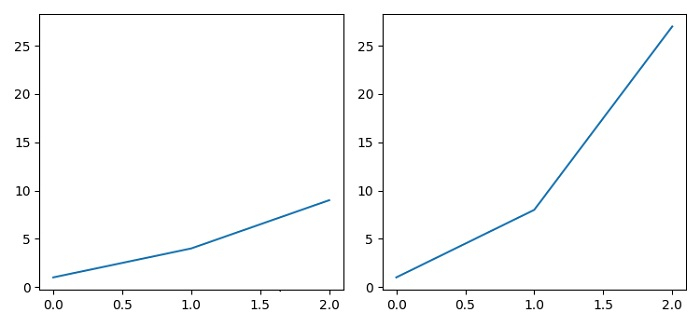# Show tick labels when sharing an axis in Matplotlib

To show the tick labels when sharing an axis, we can just use the subplot() method with sharey argument. By default, y ticklabels could be visible.

## Steps

• Set the figure size and adjust the padding between and around the subplots.

• Add a subplot to the current figure using subplot() method, where nrows=1, ncols=2 and index=1 for axis ax1.

• Plot a line on the axis 1.

• Add a subplot to the current figure, using subplot() method, where nrows=1, ncols=2 and index=2 for axis ax2.

• Plot a line on the axis 2.

• To display the figure, use show() method.

## Example

from matplotlib import pyplot as plt
plt.rcParams["figure.figsize"] = [7.50, 3.50]
plt.rcParams["figure.autolayout"] = True
ax1 = plt.subplot(1, 2, 1)
ax1.plot([1, 4, 9])
ax2 = plt.subplot(1, 2, 2, sharey=ax1)
ax2.plot([1, 8, 27])
plt.show()

## Output